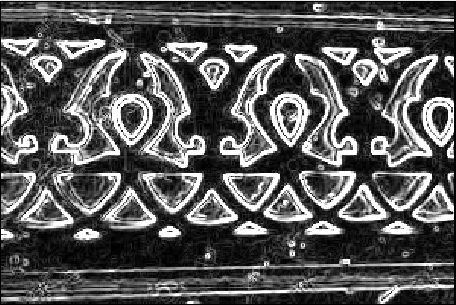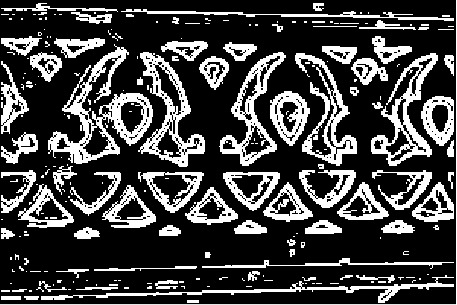# MATLAB – Image Edge Detection using Sobel Operator from Scratch

Sobel Operator: It is a discrete differentiation gradient-based operator. It computes the gradient approximation of image intensity function for image edge detection. At the pixels of an image, the Sobel operator produces either the normal to a vector or the corresponding gradient vector. It uses two 3 x 3 kernels or masks which are convolved with the input image to calculate the vertical and horizontal derivative approximations respectively –Approach:

Step 1: Input – Read an image
Step 2: Convert the true-color RGB image to the grayscale image
Step 3: Convert the image to double
Step 4: Pre-allocate the filtered_image matrix with zeros
Step 5: Define Sobel Operator Mask
Step 6: Edge Detection Process (Compute Gradient approximation and magnitude of vector)
Step 7: Display the filtered image
Step 8: Thresholding on the filtered image
Step 9: Display the edge-detected image

Implementation in MATLAB:

 % MATLAB Code | Sobel Operator from Scratch     % Read Input Image  input_image = imread('[name of input image file].[file format]');     % Displaying Input Image  input_image = uint8(input_image);  figure, imshow(input_image); title('Input Image');     % Convert the truecolor RGB image to the grayscale image  input_image = rgb2gray(input_image);     % Convert the image to double  input_image = double(input_image);     % Pre-allocate the filtered_image matrix with zeros  filtered_image = zeros(size(input_image));     % Sobel Operator Mask  Mx = [-1 0 1; -2 0 2; -1 0 1];  My = [-1 -2 -1; 0 0 0; 1 2 1];     % Edge Detection Process  % When i = 1 and j = 1, then filtered_image pixel    % position will be filtered_image(2, 2)  % The mask is of 3x3, so we need to traverse   % to filtered_image(size(input_image, 1) - 2  %, size(input_image, 2) - 2)  % Thus we are not considering the borders.  for i = 1:size(input_image, 1) - 2      for j = 1:size(input_image, 2) - 2             % Gradient approximations          Gx = sum(sum(Mx.*input_image(i:i+2, j:j+2)));          Gy = sum(sum(My.*input_image(i:i+2, j:j+2)));                            % Calculate magnitude of vector          filtered_image(i+1, j+1) = sqrt(Gx.^2 + Gy.^2);                end end    % Displaying Filtered Image  filtered_image = uint8(filtered_image);  figure, imshow(filtered_image); title('Filtered Image');     % Define a threshold value  thresholdValue = 100; % varies between [0 255]  output_image = max(filtered_image, thresholdValue);  output_image(output_image == round(thresholdValue)) = 0;     % Displaying Output Image  output_image = im2bw(output_image);  figure, imshow(output_image); title('Edge Detected Image');

Input Image –Filtered Image:Edge Detected Image:1. Simple and time efficient computation
2. Very easy at searching for smooth edges

Limitations:

1. Diagonal direction points are not preserved always
2. Sensitive to noise
3. Not very accurate in edge detection
4. Detect with thick and rough edges does not give appropriate results

My Personal Notes arrow_drop_upCheck out this Author's contributed articles.

If you like GeeksforGeeks and would like to contribute, you can also write an article using contribute.geeksforgeeks.org or mail your article to contribute@geeksforgeeks.org. See your article appearing on the GeeksforGeeks main page and help other Geeks.

Please Improve this article if you find anything incorrect by clicking on the "Improve Article" button below.

Article Tags :

Be the First to upvote.

Please write to us at contribute@geeksforgeeks.org to report any issue with the above content.